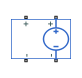# Voltage-Controlled Voltage Source

Linear voltage-controlled voltage source

## Library

Electrical Sources

•## Description

The Voltage-Controlled Voltage Source block models a linear voltage-controlled voltage source, described with the following equation:

`$V=K·\left(V\left(+\right)-V\left(-\right)\right)$`

where

 `V` Output voltage `K` Voltage gain `V(+)`,`V(-)` Voltages presented at the + and – control ports

To use the block, connect the + and – ports on the left side of the block (the control ports) to the control voltage source. The two ports on the right side of the block (the output ports) generate the output voltage. Polarity is indicated by the + and – signs.

### Variables

To set the priority and initial target values for the block variables prior to simulation, use the Initial Targets section in the block dialog box or Property Inspector. For more information, see Set Priority and Initial Target for Block Variables.

Nominal values provide a way to specify the expected magnitude of a variable in a model. Using system scaling based on nominal values increases the simulation robustness. Nominal values can come from different sources, one of which is the Nominal Values section in the block dialog box or Property Inspector. For more information, see Modify Nominal Values for a Block Variable.

## Parameters

Voltage gain K

The change in the output voltage divided by the change in the control voltage that causes it. The default value is `1`.

## Ports

The block has four electrical conserving ports. Connections + and – on the left side of the block are the control ports. The other two ports are the electrical terminals that provide the output voltage. Polarity is indicated by the + and – signs.

## Version History

Introduced in R2007a